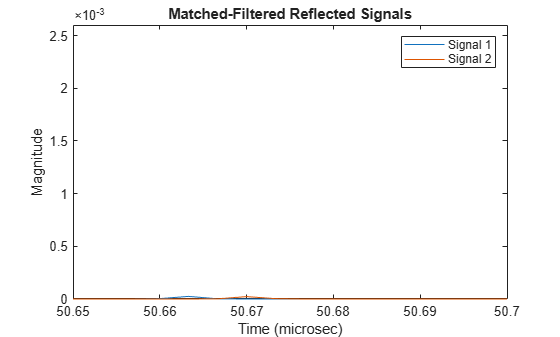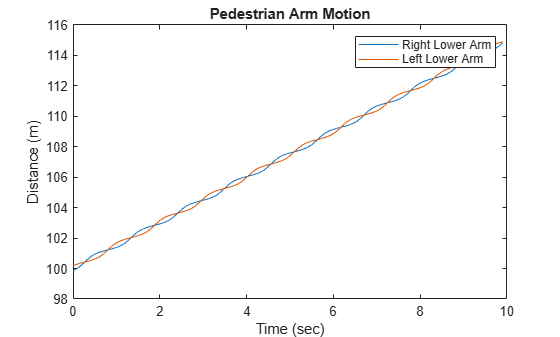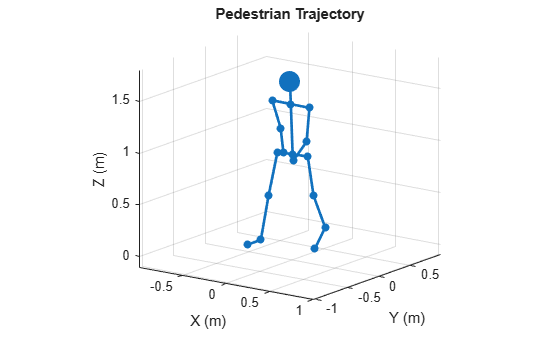# backscatterPedestrian

## Description

backscatterPedestrian creates an object that simulates signals reflected from a walking pedestrian. The pedestrian walking model coordinates the motion of 16 body segments to simulate natural motion. The model also simulates the radar reflectivity of each body segment. From this model, you can obtain the position and velocity of each segment and the total backscattered radiation as the body moves.

After creating the pedestrian, you can move the pedestrian by calling the `move` object function. To obtain the reflected signal, call the `reflect` object function. You can plot the instantaneous position of the body segments using the `plot` object function.

## Creation

### Syntax

```pedestrian = backscatterPedestrian pedestrian = backscatterPedestrian(Name,Value,...) ```

### Description

`pedestrian = backscatterPedestrian` creates a pedestrian target model object, `pedestrian`. The pedestrian model includes 16 body segments – left and right feet, left and right lower legs, left and right upper legs, left and right hip, left and right lower arms, left and right upper arms, left and right shoulders, neck, and head.

`pedestrian = backscatterPedestrian(Name,Value,...)` creates a pedestrian object, `pedestrian`, with each specified property `Name` set to the specified `Value`. You can specify additional name-value pair arguments in any order as (`Name1`,`Value1`,...,`NameN`,`ValueN`). Any unspecified properties take default values. For example,

```pedestrian = backscatterPedestrian( ... 'Height',2,'WalkingSpeed',0.5, ... 'InitialPosition',[0;0;0],'InitialHeading',90);```
models a two-meter tall woman or man moving along the positive y-axis at one-half meter per second.

## Properties

expand all

Height of pedestrian, specified as a positive scalar. Units are in meters.

Data Types: `double`

Walking speed of pedestrian, specified as a non-negative scalar. The motion model limits the walking speed to 1.4 times the pedestrian height set in the `Height` property. Units are in meters per second.

Data Types: `double`

Signal propagation speed, specified as a positive scalar. Units are in meters per second. The default propagation speed is the value returned by `physconst('LightSpeed')`. See `physconst` for more information.

Example: `3e8`

Data Types: `double`

Carrier frequency of narrowband incident signals, specified as a positive scalar. Units are in Hz.

Example: `1e9`

Data Types: `double`

Initial position of the pedestrian, specified as a 3-by-1 real-valued vector in the form of `[x;y;z]`. Units are in meters.

Data Types: `double`

Initial heading of pedestrian, specified as a scalar. Heading is measured in the xy-plane from the x-axis towards y-axis. Units are in degrees.

Data Types: `double`

## Object Functions

expand all

 `move` Position and velocity of walking pedestrian `reflect` Reflected signal from walking pedestrian `plot` Display stick figure showing the positions of all body segments of pedestrian
 `step` Run System object algorithm `release` Release resources and allow changes to System object property values and input characteristics `reset` Reset internal states of System object

## Examples

collapse all

Compute the reflected radar signal from a pedestrian moving along the x-axis away from the origin. The radar operates at 24 GHz and is located at the origin. The pedestrian is initially 100 meters from the radar. Transmit a linear FM waveform having a 300 MHz bandwidth. The reflected signal is captured at the moment the pedestrian starts to move and at two seconds into the motion.

Create a linear FM waveform and a free space channel to propagate the waveform.

```c = physconst('Lightspeed'); bw = 300.0e6; fs = bw; fc = 24.0e9; wav = phased.LinearFMWaveform('SampleRate',fs,'SweepBandwidth',bw); x = wav(); channel = phased.FreeSpace('OperatingFrequency',fc,'SampleRate',fs, ... 'TwoWayPropagation',true);```

Create the pedestrian object. Set the initial position of the pedestrian to 100 m on the x-axis with initial heading along the positive x-direction. The pedestrian height is 1.8 m and the pedestrian is walking at 0.5 meters per second.

```pedest = backscatterPedestrian( 'Height',1.8, ... 'OperatingFrequency',fc,'InitialPosition',[100;0;0], ... 'InitialHeading',0,'WalkingSpeed',0.5);```

The first call to the `move` function returns the initial position, initial velocity, and initial orientation of all body segments and then advances the pedestrian motion two seconds ahead.

`[bppos,bpvel,bpax] = move(pedest,2,0);`

Transmit the first pulse to the pedestrian. Create 16 replicas of the signal and propagate them to the positions of the pedestrian body segments. Use the `rangeangle` function to compute the arrival angle of each replica at the corresponding body segment. Then use the `reflect` function to return the coherent sum of all the reflected signals from the body segments at the pedestrian initial position.

```radarpos = [0;0;0]; xp = channel(repmat(x,1,16),radarpos,bppos,[0;0;0],bpvel); [~,ang] = rangeangle(radarpos,bppos,bpax); y0 = reflect(pedest,xp,ang);```

Obtain the position, velocity, and orientation of each body segment then advance the pedestrian motion another two seconds.

`[bppos,bpvel,bpax] = move(pedest,2,0);`

Transmit and propagate the second pulse to the new position of the pedestrian.

```radarpos = [0;0;0]; xp = channel(repmat(x,1,16),radarpos,bppos,[0;0;0],bpvel); [~,ang] = rangeangle(radarpos,bppos,bpax); y1 = reflect(pedest,xp,ang);```

Match-filter and plot both of the reflected pulses. The plot shows the increased delay of the matched filter output as the pedestrian walks away.

```filter = phased.MatchedFilter('Coefficients',getMatchedFilter(wav)); ymf = filter([y0 y1]); t = (0:size(ymf,1)-1)/fs; plot(t*1e6,abs(ymf)) xlabel('Time (microsec)') ylabel('Magnitude') title('Match-Filtered Reflected Signals') legend('Signal 1','Signal 2')```Zoom in and show the time delays for each signal.

```plot(t*1e6,abs(ymf)) xlabel('Time (microsec)') ylabel('Magnitude') title('Matched-Filtered Reflected Signals') axis([50.65 50.7 0 .0026]) legend('Signal 1','Signal 2')```Create a pedestrian object. Set the initial position of the pedestrian to 100 m on the x-axis with initial heading along the positive x-direction. The pedestrian height is 1.8 m and the pedestrian is walking at 1.5 meters per second.

```fc = 24.0e9; pedest = backscatterPedestrian( 'Height',1.8, ... 'OperatingFrequency',fc,'InitialPosition',[100;0;0], ... 'InitialHeading',0,'WalkingSpeed',1.5);```

Obtain and plot the detailed motion of the right and left lower arms of the pedestrian by capturing their positions every 1/10th of a second.

```blla = zeros(3,100); brla = blla; t = zeros(1,100); T = .1; for k = 1:100 [bppos,bpvel,bpax] = move(pedest,T,0); blla(:,k) = bppos(:,9); brla(:,k) = bppos(:,10); t(k) = T*(k-1); end plot(t,brla(1,:),t,blla(1,:)) title('Pedestrian Arm Motion') xlabel('Time (sec)') ylabel('Distance (m)') legend('Right Lower Arm','Left Lower Arm')```Display the motion of a pedestrian walking a square path. Create the pedestrian using a `backscatterPedestrian` object with default values except for height which is 1.7 meters. Advance and display the pedestrian position every 3 milliseconds. First, the pedestrian moves along the positive x-axis, then along the positive y-axis, along the negative x-axis, and finally along the negative y-axis to return to the starting point.

```ped = backscatterPedestrian('Height',1.7); dt = 0.003; N = 3600; for m = 1:N if (m < N/4) angstep = 0.0; end if (m >= N/4) angstep = 90.0; end if (m >= N/2) angstep = 180.0; end if (m >= 3*N/4) angstep = 270.0; end move(ped,dt,angstep); plot(ped) end```Victor Chen, The Micro-Doppler Effect in Radar, Artech House, 2011.

 Ronan Boulic, Nadia Magnenat-Thalmann, Daniel Thalmann, A Global Human Walking Model with Real-time Kinematic Personification, The Visual Computer: International Journal of Computer Graphics, Vol. 6, Issue 6, Dec 1990.